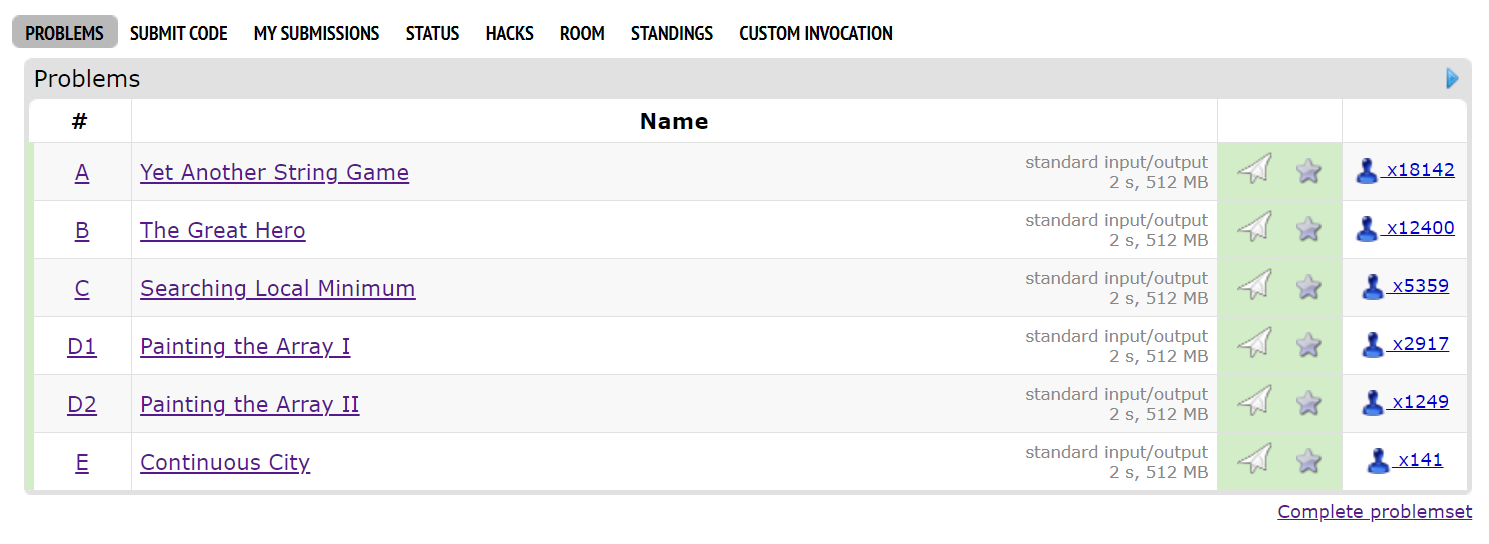# Codeforces Round #700 (Div. 2) 题解Round 700 Div.2给人的感觉是手速场，基本上没有什么很需要思考的点。

### A. Yet Another String Game

#include<bits/stdc++.h>
using namespace std;
typedef long long ll;
const int maxn=1e5+7;

bool done;

int main(){
ios::sync_with_stdio(false);cin.tie(0);cout.tie(0);
int t;
string s;
cin>>t;
while(t--){
cin>>s;
int len=s.length();
memset(done,0,sizeof(done));
for(int i=0;i<len;++i){
for(int j=0;j<len;++j){
if(!done[j]){
done[j]=true;
if(i&1){
s[j] = (s[j] == 'z' ? 'y' : 'z');
}else {
s[j] = (s[j] == 'a' ? 'b' : 'a');
}
break;
}
}
}
cout<<s<<"\n";
}
}


### B. The Great Hero

#include<bits/stdc++.h>
using namespace std;
typedef long long ll;
const int maxn=1e5+7;

long long a[maxn],b[maxn],loss[maxn],id[maxn];

int main(){
ios::sync_with_stdio(false);cin.tie(0);cout.tie(0);
long long t,A,B,n;
cin>>t;
while(t--){
cin>>A>>B>>n;
for(int i=1;i<=n;++i){
cin>>a[i];
}
for(int i=1;i<=n;++i){
cin>>b[i];
}
ll sum=0;
for(int i=1;i<=n;++i){
id[i]=i;
loss[i]=(b[i]+A-1)/A*a[i];
sum+=loss[i];
}
sort(id+1,id+1+n,[](int i,int j){
return a[i]<a[j];
});// 没必要排序,只需要找最大的a[i],懒得改了
if(sum-B<a[id[n]]){
cout<<"YES\n";
}else{
cout<<"NO\n";
}
}
}


### C. Searching Local Minimum

#include<bits/stdc++.h>
using namespace std;
typedef long long ll;
const int maxn=1e5+7;

int arr[maxn];

int main(){
ios::sync_with_stdio(false);cin.tie(0);cout.tie(0);
int l,r,mid,n;
cin>>n;
l=1,r=n;
arr=arr[n+1]=1e9+7;
if(n<=100){
for(int i=1;i<=n;++i){
cout<<"? "<<i<<endl;
cin>>arr[i];
}
for(int i=1;i<=n;++i){
if(arr[i-1]>arr[i]&&arr[i]<arr[i+1]){
cout<<"! "<<i<<endl;
return 0;
}
}
}
int cnt=100;
while(l!=r){
if(r-l<=cnt){
for(int i=l;i<=r;++i){
cout<<"? "<<i<<endl;
cin>>arr[i];
}
for(int i=l;i<=r;++i){
if(arr[i-1]>arr[i]&&arr[i]<arr[i+1]){
cout<<"! "<<i<<endl;
return 0;
}
}
}
mid=(l+r)>>1;
cout<<"? "<<mid<<endl;--cnt;
cin>>arr[mid];
cout<<"? "<<mid+1<<endl;--cnt;
cin>>arr[mid+1];
if(arr[mid]>arr[mid+1]){
l=mid+1;
}else{
r=mid;
}
}
cout<<"! "<<l<<endl;
}


### D1. Painting the Array I

1. 放进两个栈里都不会发生合并。此时要往后多考虑一步第$a_{i+1}$的安排，如果$a_{i+1}$和其中某一个栈顶部的元素相同我们就选择把$a_{i}$放在这里堵住他，可以让后面的$a_{i+1}$不被合并。
2. 一个栈可以合并一个栈不能合并，或者两个栈都能合并。此时尽量选不能自身的就可以，因为即使能像上一步的一样救一个元素也只能救$a_{i+1}$，但是自身被合并了，1换1等于没做。

#include<bits/stdc++.h>
using namespace std;
typedef long long ll;
const int maxn=1e5+7;

int arr[maxn],dp[maxn],cnt[maxn],top;
vector<int> a1,a2;

int main(){
ios::sync_with_stdio(false);cin.tie(0);cout.tie(0);
int n,ans=0;
cin>>n;
for(int i=1;i<=n;++i) cin>>arr[i];
for(int i=1;i<=n;++i){
if(i<n&&(a1.empty()||*a1.rbegin()!=arr[i])&&(a2.empty()||*a2.rbegin()!=arr[i])){
if(!a1.empty()&&*a1.rbegin()==arr[i+1]){
a1.push_back(arr[i]);
}else{
a2.push_back(arr[i]);
}
}
else if(a1.empty()||*a1.rbegin()!=arr[i]){
a1.push_back(arr[i]);
}
else if(a2.empty()||*a2.rbegin()!=arr[i]){
a2.push_back(arr[i]);
}
}
ans=max(ans,(int)a1.size()+(int)a2.size());
cout<<ans;
}


### D2. Painting the Array II

1. 其中某一个栈可以合并掉$a_i$。此时就直接毫不犹豫地合并就完事了，因为合并了会少一个数字而且完全影响不到后面的其他数字。
2. 两个栈都不能合并$a_i$，那么我们操作时就需要选择保留一个“合并潜力”更大地栈顶元素。衡量这个“合并潜力”便用到了上面的分析：选择合并后一个相同数字所花费代价更小的那个。正式地说，若令$lst[i]$表示第$i$个位置之后的第1个与 $a_i$ 相同的元素位置，那么我们会选择保留 $c=\argmin{lst[Stack[i].top]}, i={1,2}$ ，而将 $a_i$ 放到另一个栈里。

#include<bits/stdc++.h>
using namespace std;
typedef long long ll;
const int maxn=1e5+7;

int arr[maxn],lst[maxn],pos[maxn],dp[maxn],cnt[maxn],top;
vector<pair<int,int> > a1,a2;

int main(){
ios::sync_with_stdio(false);cin.tie(0);cout.tie(0);
int n,ans=0;
cin>>n;
for(int i=1;i<=n;++i){
cin>>arr[i];
}
cnt[top=1]=1;
for(int i=2;i<=n;++i){
if(arr[top]!=arr[i]){
arr[++top]=arr[i];
cnt[top]=1;
}else{
cnt[top]++;
}
}
memset(pos,0x3f,sizeof(pos));
for(int i=top;i>=1;--i){
lst[i]=pos[arr[i]];
pos[arr[i]]=i;
}

for(int i=1;i<=top;++i){
if(!a1.empty() && (a1.rbegin()->second==arr[i])){
a1.rbegin()->first=i;
}else if(!a2.empty() && (a2.rbegin()->second==arr[i])){
a2.rbegin()->first=i;
}else if(a1.empty()){
a1.push_back({i,arr[i]});
}else if(a2.empty()){
a2.push_back({i,arr[i]});
}else{
if(lst[a1.rbegin()->first]<lst[a2.rbegin()->first]){
a2.push_back({i,arr[i]});
}else{
a1.push_back({i,arr[i]});
}
}
}
cout<<a1.size()+a2.size();
}


### E. Continuous City

1. 从点 $1$ 到 点 $n$ 的所有路径的长度必须在 $[L,R]$之间
2. 对于每一个 $L\le d \le R$，有且仅有一条路径满足其长度等于$d$.

“5 3 1 1“ 这样一个分解集。类似二进制的思想，把这些分解出来的值当作某个进制的权值，则任意一个$[L,R]$之间的整数都可以唯一的由该分解集的一个子集表示。例如$1-10$中，$4=3+1$，$6=5+1$，$7=5+1+1$，这些都是上面分解集的子集，且这种表示是唯一的。

1. 初始边，直接把点1到点2之间的有向边设置为 $L-1$ ，并把$2$设置为初始点（当$L1$的时候则不需要此步，直接令$1$为初始点）。
2. 将从初始点开始的之后的第 $i$ 个点与比他更小的每个点都连接一条入边，且对于该点的所有入边权值均为分解中从小到大的第 $i$ 个元素。另外，为了保证路径的唯一性，若在分块的过程中有某一次分出的两个块大小相同，则只选取其中一个与初始点相连。例如分解出来
“5 3 1 1“，则只有 “3 1 1“ 会跟初始点直接相连，这样就不会出现有两条长度为5的路径的情况了。
3. 所有的点都向点 $n$ 连一条长度为 $1$ 的边。

#include<bits/stdc++.h>
using namespace std;
typedef long long ll;
const int maxn=1e5+7;

int g;
vector<int> vec;

int main(){
ios::sync_with_stdio(false);cin.tie(0);cout.tie(0);
int l,r,cntn=0,cntm=0,base=1;
cin>>l>>r;
if(l>1){
g=l-1;cntm++;
base=2;
}
if(r==l){
cout<<"YES\n"<<"2 1\n"<<"1 2 "<<l<<"\n";
return 0;
}else if(l+1==r){
cout<<"YES\n"<<"3 2\n"<<"1 2 "<<l<<"\n"<<"2 3 1\n";
return 0;
}
for(int diff=r-l;diff;diff>>=1){
if(diff&1){
vec.push_back(diff/2+1);
}else{
vec.push_back(diff/2);
}
}
sort(vec.begin(),vec.end());
int len=vec.size(),presum=0;
cntn=len+1+base;
g[base][cntn]=1;cntm++;
for(int i=0;i<len;++i){
int to=base+i+1;
for(int j=(presum==vec[i]?0:-1);j<i;++j){
int from=base+j+1;
g[from][to]=vec[i];cntm++;
}
g[to][cntn]=1;cntm++;
presum+=vec[i];
}
cout<<"YES\n"<<cntn<<" "<<cntm<<"\n";
for(int i=1;i<cntn;++i){
for(int j=i+1;j<=cntn;++j){
if(g[i][j]){
cout<<i<<" "<<j<<" "<<g[i][j]<<"\n";
}
}
}
}